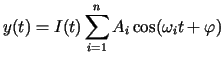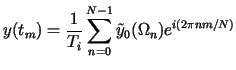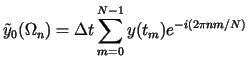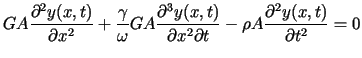Computational & Technology Resources
an online resource for computational,
engineering & technology publications
Civil-Comp Proceedings
ISSN 1759-3433
CCP: 73
PROCEEDINGS OF THE EIGHTH INTERNATIONAL CONFERENCE ON CIVIL AND STRUCTURAL ENGINEERING COMPUTING
Edited by: B.H.V. Topping
Paper 125

Effect of Base Level to Internal Forces of a Structure in case of Earthquake

J. Györgyi and S. Ádány

Department of Structural Mechanics, Budapest University of Technology and Economics, Hungary

Full Bibliographic Reference for this paper
J. Györgyi, S. Ádány, "Effect of Base Level to Internal Forces of a Structure in case of Earthquake", in B.H.V. Topping, (Editor), "Proceedings of the Eighth International Conference on Civil and Structural Engineering Computing", Civil-Comp Press, Stirlingshire, UK, Paper 125, 2001. doi:10.4203/ccp.73.125
Keywords: earth-quake analysis, soil-structure interaction, damping effect.

Summary
Seismic analysis of structures is based on the ground motion which is normally given at the ground surface and defined by the response spectra in the horizontal and vertical directions. On the basis of these response spectra it is possible to generate artificial accelerograms which correspond to the response spectrum and allow to calculate the internal forces of the structure by taking into consideration the soil interaction , which, due to damping, can have advantageous effect to the structure. This analysis, however, can be performed in the frequency domain only. In this procedure the artificial time-displacement function is given as the sum of certain number of harmonic components:(125.1)

where I(t) is the intensity function to limit the length of the earth-quake to the required time. By applying discrete Fourier transformation, the displacement function can be decomposed into harmonic components:(125.2)

where(125.3)

These harmonic components correspond to the motion at the ground level. However, it is possible to consider the effect of soil damping, which requires the solution of the differential equation for shear waves to each component, as follows:(125.4)

From the solution of this equation the reduced amplitude of each component can be determined in the base level.

From the calculated harmonic components further analysis of the structure can be performed, or the displacement function and the response spectrum at the base level can be established by applying inverse Fourier transformation. This response spectrum differs from the one at ground surface, even its shape is different, since the frequency-independent internal damping of the soil results is higher reduction of the amplitudes for waves with higher frequency (and shorter wavelength) than the ones with lower frequency (longer wavelength).

For each structure the distribution of eigen-frequencies is different, due to the difference in the type and stiffness properties of the structure. Thus, the importance of reduction of response spectrum to the base level will be different for different structures.

In the paper the results of some numerical simulation is presented which is performed on tall "tower-like" and stiffer "box-like" buildings. In case of the first group these are the eigen-shapes belonging to the lower eigen-frequencies which have essential role in the internal force calculation, while for the second group the important eigen-frequencies are higher and fall into a wider range. Consequently, the reduction of the ground motion has more significant effect for this latter type of structures.

In the paper the internal forces and displacements are calculated for different types of structures and with different base levels. The results are compared to the ones calculated from the unreduced ground motion.

References
1
Idriss, I. M. et al: "Analyses for soil-structure interaction effects for nuclear power plants" ASCE, New York, 1979.

purchase the full-text of this paper (price £20)